### Home > PC > Chapter 4 > Lesson 4.1.2 > Problem4-26

4-26.
1. Rewrite each of the following logarithmic expressions in terms of a single log. Homework Help ✎

1. log M + 2 log N

2.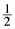(log P − log Q)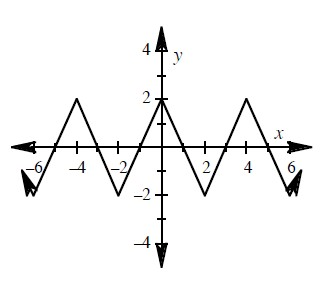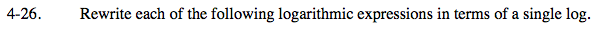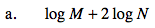Bring the 2 up as an exponent first. Then combine the two logs into one.

log(MN2)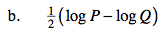Combine the logs first into one log. Be careful with the sign.

$\text{Bring the }\frac{1}{2}\text{ up as an exponent for both logs.}$

$\text{log}\sqrt{\frac{P}{Q}}$